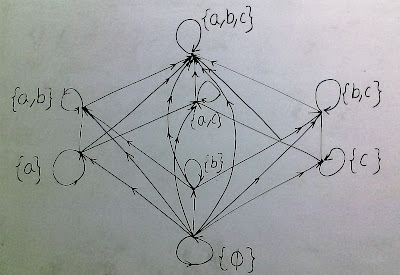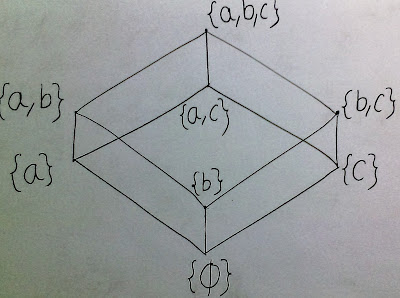### Hasse diagram

HASSE DIAGRAM

Hasse Diagram: Hasse diagram generally used to represent partial order relations in equivalent but in simpler forms by removing non critical parts of the relation.

For example:Below diagram represent a partial order relation.

This can relation can be modify as-
1. Remove all loops.
2. Remove all arrows that can be inferred from transitivity.

Than above diagram represented as-Above diagram is known as Hasse diagram for a partial order relation.

Problems based on above study:

Prob. Draw the Hasse Diagram of D60 (divisors of 60).
Solution.

Prob. What is Hasse diagram? Draw the Hasse diagram of the set D30 of positive integral divisor of 30 with relation ‘1’.

Solution.

Prob. Let A = ( a, b, c, d} and P(A) its power set. Draw Hasse diagram of [P(A), ].

Solution.

Prob. Let A = {2, 3, 6, 12, 24, 36} and the relation  be such that a ≤ b, if a divides b. Draw the Hasse diagram of (A ≤).
Solution.